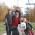Mixture problems - algebra 1

I am hoping you can help me. I can not remember how to solve mixture problems and how to set them up. Examples are as follows:
A merchant made a mixture of 150lb. of tea worth \$109.50 by mixing tea worth \$1.25 a pound with tea worth \$.65 a pound. How many pounds of each kind did he use?

Organizing the information in a table or chart is usually very helpful in dealing with mixture problems. Other than that, it helps to study several examples and practice solving them yourself. After a while, it gets easier and patterns begin to emerge.

The first problem has two unknowns. Let x be the amount of more expensive tea, and y the amount of the cheaper tea (in pounds).

In our table, we will look at the amounts of tea (in pounds), price per pound, AND the amount the tea is worth, which is (the amount) times (the price).

amount | price per lb | worth
-------------------------------------
x | \$1.25 | 1.25x
-------------------------------------
y | \$0.65 | 0.65y
-------------------------------------

Then we add one more row to the table that has to do with the MIXTURE, or the total.

amount | price per lb | worth
-------------------------------------
x | \$1.25 | 1.25x
-------------------------------------
y | \$0.65 | 0.65y
-------------------------------------
150 lb | ?? | \$109.50

Now we get our equations. First of all, x + y = 150. And secondly, 1.25x + 0.65y = 109.50.

This gives you a system of two linear equations to solve, using any standard technique. For example, you can solve from the first that y = 150 − x and substitute that into the second.

The solution is: x = 20, y = 130.

Check: we have 20 lbs of tea costing \$1.25 per pound, so it is worth \$25.
We have 130 lbs of tea costing \$0.65 per pound, so it is worth \$84.50. Total worth is \$109.50. It checks.

A pharmacist has 10 oz. of salt and water of which 4 oz. are salt. How may ounces of water must he add so that 5% of the new solution is salt.

This is a very typical (and routine) problem from algebra 1 textbooks. Here, our table will have one row for the original situation, and another for the final situation. We are checking the amounts of salt and water, and then the total amount.

| salt | water | total
-------------------------------------
1st situation | 4 | 6 | 10
-------------------------------------
2nd situation | 4 | ? | ?
-------------------------------------

The KEY is that there is no salt added, only water. Our unknown is the amount of water added.

| salt | water | total
---------------------------------------
1st situation | 4 | 6 | 10
---------------------------------------
2nd situation | 4 | 6 + x | 10 + x
---------------------------------------

The equation is gotten from the statement that 5% of the new solution is salt. This means that 5% of the total (which is 10 + x) is salt (which we know to be 4 oz).

0.05(10 + x) = 4.

0.5 + 0.05x = 4
0.05x = 3.5
x = 3.5 / 0.05 = 70.

He needs to add 70 oz of water.

In 110 lb. of an alloy of tin and copper, the amount of tin was 5lb. less than 1/3 that of the copper. How may pounds of tin were there?

Again, we organize this into a table:

| tin | copper | total
--------------------------------------
| t | c | 110
---------------------------------------

We have TWO unknowns: the amount of tin and the amount of copper. Right there we get one equation: t + c = 110. The statement "the amount of tin was 5lb. less than 1/3 that of the copper" allows us to build another equation relating t and c.

t = (1/3)c - 5

Again, a system of equations. Since t is expressed in terms of c in the equation above, I use that to substitute to the first equation:

(1/3)c - 5 + c = 110

(4/3)c = 115

c = 115 * 3 / 4

c = 86.25.

But it asked for t, so t is 110 - 86.25 = 23.75 lb.

I hope these examples were helpful in dealing with "mixture" type problems in algebra.muguet said…
I invite you to "pick up" The "One lovely blog award" on my blog.

http://famillehamel.blogspot.com/

Sorry...I'm francophone and my english is so, so...muguet said…
Sorry...see november 5 on my blogTables and charts do help but I found interesting is after some students are taught charts or tables, they could not directly go to system of equations to just set up equations and to work out problems. Their thought are so limited to tables now.

I demonstrated on how to solve it without using tables or charts, then some students found it is "easier" to do it by just thinking the way on how to solve it.

"Tools" actually confine some studnet's natural thinking ability to solve problem, this is a very typical example.